# Subtracting Rational Numbers

Subtracting Rational Numbers, deals with various concepts which are as under:-

• Subtracting Rational Numbers When Denominators are Equal
• Subtracting Rational Numbers When Denominators are Unequal

## Subtracting Rational Numbers When Denominators are Equal

Question 1

Subtract:

13/7 – 8/7

Explanation

Difference of Rational Numbers when Denominators are equal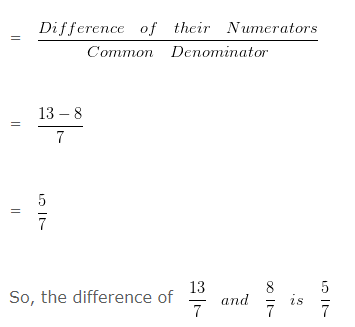Question 2

Subtract:

34/13 – 12/13

Explanation

Difference of Rational Numbers when Denominators are equal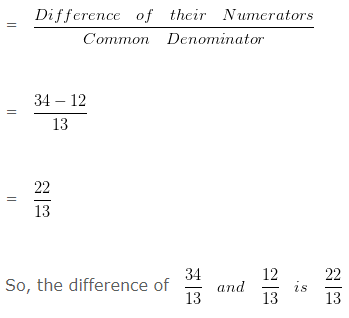## Subtracting Rational Numbers When Denominators are Unequal

Question 3

Subtract:

3/4 – 2/7

Explanation

To subtract two Rational Numbers with different Denominators, first we will find the LCM of both the Denominators.

LCM of 4 and 7 is 28

Now we would divide such LCM by Denominator of first number and the result would be multiplied with both the numerator and denominator of such number

Divide LCM by the Denominator of first number

28 ÷ 4 = 7

We have to multiply , both the Numerator and Denominator of 3/4 by the quotient i.e, 7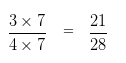Divide LCM by the Denominator of second number

28 ÷ 7 = 4

We have to multiply , both the Numerator and Denominator of 2/7 by the quotient i.e, 4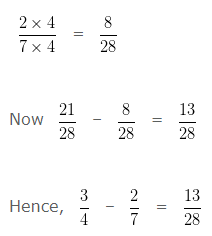Question 4

Subtract:

5/4 – 3/5

Explanation

To subtract two Rational Numbers with different Denominators, first we will find the LCM of both the Denominators.

LCM of 4 and 5 is 20

Now we would divide such LCM by Denominator of first number and the result would be multiplied with both the numerator and denominator of such number

Divide LCM by the Denominator of first number

20 ÷ 4 = 5

We have to multiply , both the Numerator and Denominator of 5/4 by the quotient i.e, 5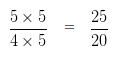Divide LCM by the Denominator of second number

20 ÷ 5 = 4

We have to multiply , both the Numerator and Denominator of 3/5 by the quotient i.e, 4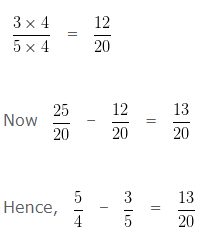## More Examples on Rational Numbers

Question 5

Find the number that should be added to -7/4 to make it 9/8?

Explanation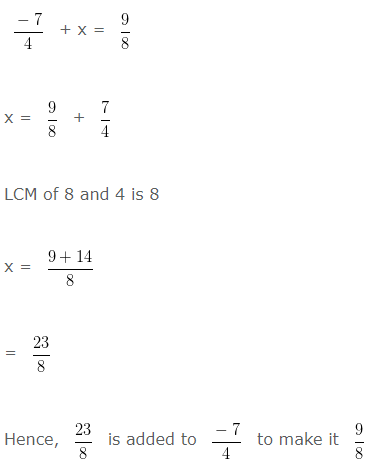Question 6

Find the number that should be added to -5/6 to make it 6/5?

Explanation

Let x be the number, which needs to be added to -5/6 to get 6/5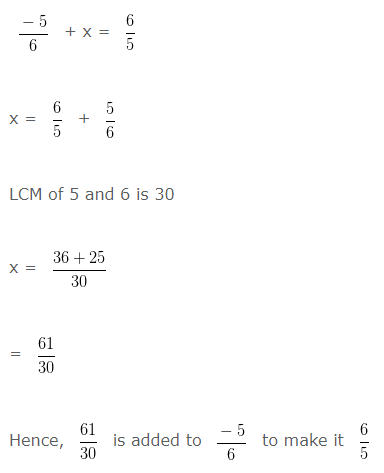Question 7

The sum of two Rational Number is -2 . If one of them is 3/2 then, other Rational Number is?

Explanation

Let, the other Rational Number be x

We are given that the sum of the two Rational Numbers is = -2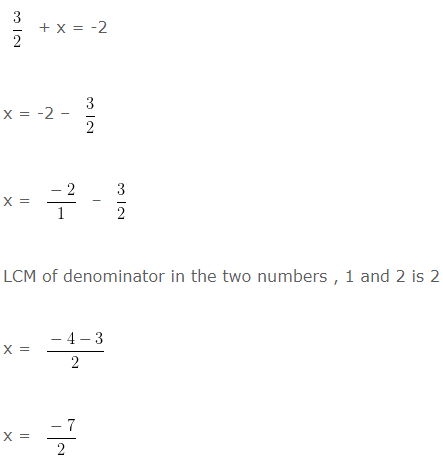Hence, the other Rational Number is -7/2

Question 8

The sum of two Rational Number is -3/4 . If one of them is -4/12 then, other Rational Number is?

Explanation

Let, the other Rational Number be x

We are given that the sum of the two Rational Numbers is = -3/4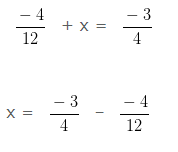LCM of denominator in the two numbers , 4 and 12 is 12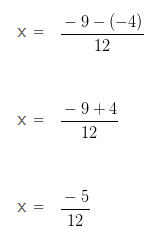Hence, the other Rational Number is -5/12